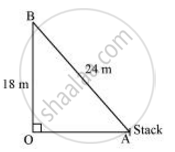# A guy wire attached to a vertical pole of height 18 m is 24 m long and has a stake attached to the other end. How far from the base of the pole should the stake be driven so that the wire will be taut? - Mathematics

A guy wire attached to a vertical pole of height 18 m is 24 m long and has a stake attached to the other end. How far from the base of the pole should the stake be driven so that the wire will be taut?

#### SolutionLet OB be the pole and AB be the wire.

By Pythagoras theorem,

AB2 = OB2 + OA2

(24m)2 = (18m)2 + OA2

OA2 = (576 - 324)m2 = 25 m2

OA2 = sqrt252

Therefore, the distance from the base is 6sqrt7 m

Concept: Right-angled Triangles and Pythagoras Property
Is there an error in this question or solution?

#### APPEARS IN

NCERT Class 10 Maths
Chapter 6 Triangles
Exercise 6.5 | Q 10 | Page 151
RD Sharma Class 10 Maths
Chapter 7 Triangles
Exercise 7.7 | Q 20 | Page 121